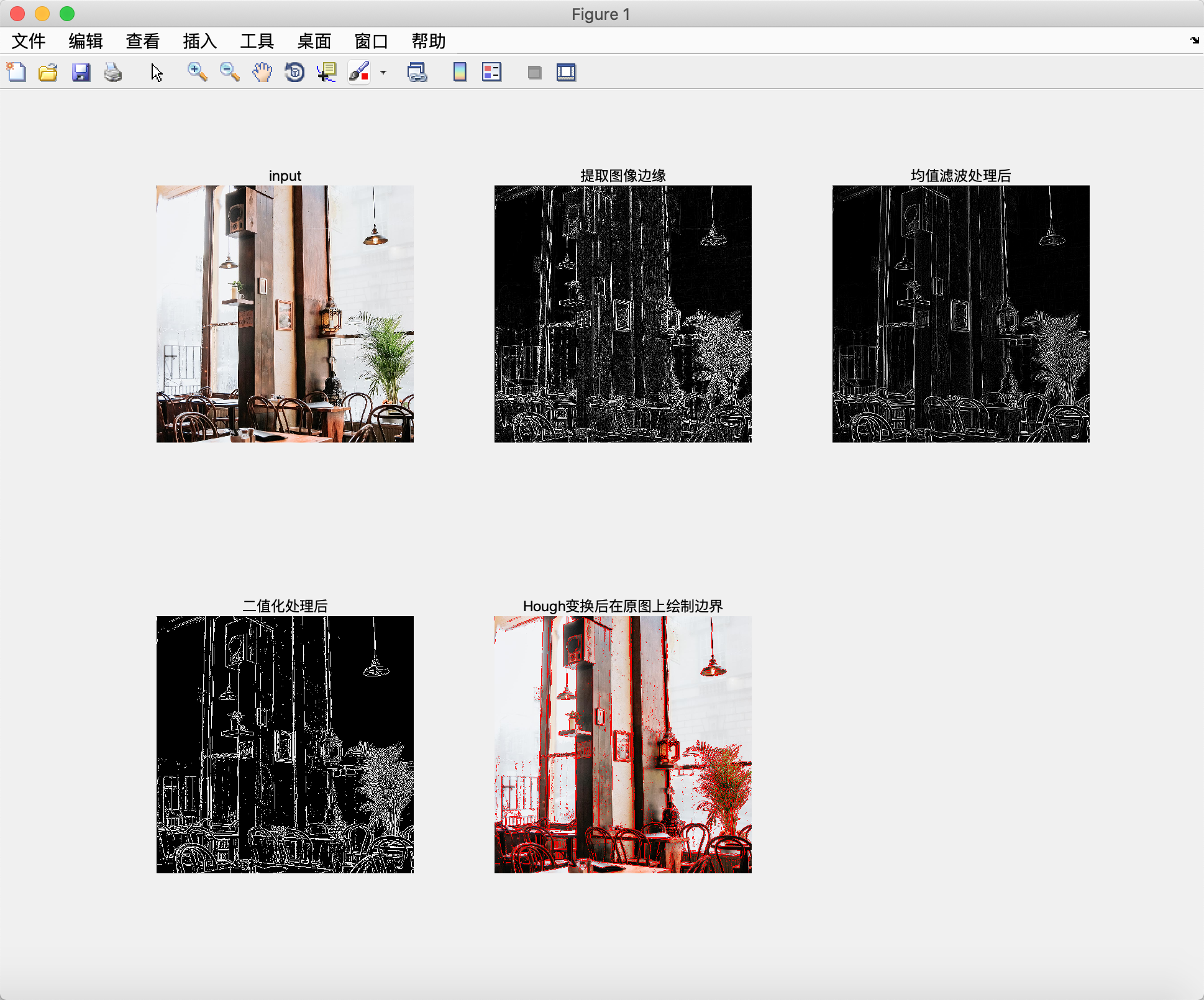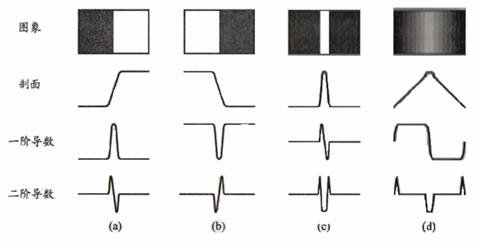2017-10-22 13:18:23 u013165921 阅读数 7155
• ###### MATLAB图像处理

全面系统的学习MATLAB在图像处理中的应用

19935 人正在学习 去看看 魏伟

## 点检测

``````f = imread('test_pattern_with_single_pixel.tif');
w = [-1 -1 -1;-1 8 -1;-1 -1 -1];                    % 点检测掩模
g = abs(imfilter(double(f),w));
T = max(g(:));
g = g>=T;
subplot(1,2,1);imshow(f);title('原图像');
subplot(1,2,2);imshow(g);title('点检测');
``````## 线检测``````f = imread('wirebond_mask.tif');        % 图像大小：486×486
w = [2 -1 -1;-1 2 -1;-1 -1 2];          % -45°方向检测线
g = imfilter(double(f),w);
gtop = g(1:120,1:120);                  % 左上角区域
gtop = pixeldup(gtop,4);                % 通过复制像素将图像扩大gtop*4倍
gbot = g(end-119:end,end-119:end);      % 右下角区域
gbot = pixeldup(gbot,4);
g1 = abs(g);                             % 检测图的绝对值
T = max(g1(:));
g2 = g1>=T;

subplot(3,2,1);imshow(f);title('原图像');
subplot(3,2,2);imshow(g,[]);title('线检测-45°方向');
subplot(3,2,3);imshow(gtop,[]);title('左上角区域');
subplot(3,2,4);imshow(gbot,[]);title('右下角区域');
subplot(3,2,5);imshow(g1,[]);title('图像绝对值');
subplot(3,2,6);imshow(g2);title('g>=T');``````使用Hough变换作线检测：

Hough变换是一种寻找并链接图像中线段的处理方式，用其进行线检测和链接的第一步是峰值检测。

## 边缘检测``````f = imread('bld.tif');
[g_sobel_default,ts] = edge(f,'sobel');
[g_log_default,tlog] = edge(f,'log');
[g_canny_default,tc] = edge(f,'canny');

g_sobel_best = edge(f,'sobel',0.05);
g_log_best = edge(f,'log',0.003,2.25);
g_canny_best = edge(f,'canny',[0.04 0.10],1.5);

subplot(3,2,1);imshow(g_sobel_default);title('默认sobel');
subplot(3,2,2);imshow(g_sobel_best);title('默认log');
subplot(3,2,3);imshow(g_log_default);title('默认canny');
subplot(3,2,4);imshow(g_log_best);title('最佳sobel');
subplot(3,2,5);imshow(g_canny_default);title('最佳log');
subplot(3,2,6);imshow(g_canny_best);title('最佳canny');``````2017-09-22 19:41:43 u011271038 阅读数 522
• ###### MATLAB图像处理

全面系统的学习MATLAB在图像处理中的应用

19935 人正在学习 去看看 魏伟

# 原理

## 梯度算子其中，## Roberts算子## Prewitt算子## Sobel算子## canny检测

1)用高斯平滑处理
2)算出梯度和梯度方向
3)对梯度值进行非极大值抑制
4)用双阈值算法检测和连接边缘2019-12-14 23:07:41 qq_43571150 阅读数 74
• ###### MATLAB图像处理

全面系统的学习MATLAB在图像处理中的应用

19935 人正在学习 去看看 魏伟

## Matlab-图像分割与边缘检测实验-Hough线检测Matlab代码👇

``````clear all;
origin_img = I;			%复制彩色原图以最后对边缘上色
img = rgb2gray(I);        %将图像转换成为灰度图
img = im2double(img);       %将图像转换成为double类型

[m,n]=size(img);		% 得到图像大小行m，列n
for i=3:m-2
for j=3:n-2		% 计算区域从图像(3,3)开始，到(m-2,n-2)结束
% LoG算子粗提取图像边缘
log_margin(i,j) = -img(i-2,j)-img(i-1,j-1)-2*img(i-1,j)-img(i-1,j+1)-img(i,j+2)-2*img(i,j-1)+16*img(i,j)-2*img(i,j+1)-img(i,j+2)-img(i+1,j-1)-2*img(i+1,j)-img(i+1,j+1)-img(i+2,j);
end
end

% 均值滤波处理
[m,n]=size(log_margin);
for i=2:m-1
for j=2:n-1
% LoG算子粗提取边缘后，进行均值滤波去除噪声（3x3范围）
smooth(i,j) = log_margin(i-1,j-1)+log_margin(i-1,j)+log_margin(i-1,j+1)+log_margin(i,j-1)+log_margin(i,j)+log_margin(i,j+1)+log_margin(i+1,j-1)+log_margin(i+1,j)+log_margin(i+1,j+1);
smooth(i,j) = smooth(i,j)/9;
end
end

% 二值化处理
binary_img = im2uint8(smooth);  % 将均值滤波后的图像转换为uint8类型图像，即图像色彩范围[0,255]
[m,n]=size(binary_img);
for i=1:m
for j=1:n
if binary_img(i,j)>50   % 设置二值化的阈值为80
binary_img(i,j) = 255;  % 超过阈值的设为白点
else
binary_img(i,j) = 0;    %小于阈值的设为黑点
end
end
end

% Hough变换检测，使用(a,p)参数空间（极坐标），a∈[0,180]，p∈[0,2d]
angle = 180;		% 极坐标角度的值为0~180度
distance = round(sqrt(m^2+n^2));		% 图像对角线长度最大值distance，从而确定p的取值范围为[-distance,distance]
number_ap = zeros(angle,2*distance);		% 存储每个检测点(a,p)的个数
coordinate = cell(angle,2*distance);		% 用元胞数组存储每个检测点的坐标(i,j)
% 投票法检测边缘白点
for i = 1:m
for j = 1:n		% 遍历二值化图像的每个点
if(binary_img(i,j) == 255)		% 仅对于每一个白点边缘点进行检测
for k = 1:angle
% 对每个点从1~180度遍历，取得经过该点的所有直线的p值（产生选民）
p = round(i*cos(pi*k/180)+j*sin(pi*k/180));
% 若p大于0，则将点存储在坐标集合(distance,2*distance)空间（投票存储器），该集合点的极坐标θ∈[0,90]
if(p > 0)
number_ap(k,distance+p) = number_ap(k,distance+p)+1;		% 符合直线的点(a,p)数量加1
coordinate{k,distance+p} = [coordinate{k,distance+p},[i,j]];		% 在点集合中存储该点坐标(i,j)
else
ap = abs(p)+1;		% 若p小于0，则将点存储在坐标集合(0,distance)空间，该集合点的极坐标θ∈[90，180]
number_ap(k,ap) = number_ap(k,ap)+1;		% 符合直线的点(a,p)数量加1
coordinate{k,ap} = [coordinate{k,ap},[i,j]];		% 在点集合中存储该点坐标(i,j)
end
end
end
end
end
% 绘制图像边缘
for i = 1:angle
for j = 1:distance*2            % 遍历累加器number_ap和点坐标coordinate

if(number_ap(i,j)>30)		% 提取直线的阈值
point = coordinate{i,j};		%提取对应点坐标
[m,n] = size(point);
%对满足阈值条件的累加器单元中(a,p)对应的所有点进行操作
for k = 1:number_ap(i,j)
origin_img(point(2*k-1),point(2*k),1) = 255;		% 每个点R分量=255，G分量=0，B分量=0
origin_img(point(2*k-1),point(2*k),2) = 0;
origin_img(point(2*k-1),point(2*k),3) = 0;		% 为满足阈值要求的直线上的点赋红色
end
end

end
end
subplot(2,3,1);imshow(I);title('input');
subplot(2,3,2);imshow(log_margin);title('提取图像边缘');
subplot(2,3,3);imshow(smooth);title('均值滤波处理后');
subplot(2,3,4);imshow(binary_img);title('二值化处理后');
subplot(2,3,5);imshow(origin_img);title('Hough变换后在原图上绘制边界');
imwrite(origin_img,'05 Hough变换 边界30.jpg');

``````

2018-04-01 19:44:05 iefenghao 阅读数 420
• ###### MATLAB图像处理

全面系统的学习MATLAB在图像处理中的应用

19935 人正在学习 去看看 魏伟

## .1 边缘检测技术概况

计算机视觉处理可以看作是为了实现某一任务从包含有大量的不相关的信息中抽出对我们有用的信息。这就意味着要扔掉一些不必要的信息，所以我们需要尽可能利用物体的不变性质。而边缘就是最重要的不变性质:光线的变化显著地影响了一个区域的外观，但是不会改变它的边缘。最重要的是人的视觉系统也是对边缘最敏感的。边缘是图像的最基本特征。所谓边缘，是指图像中灰度发生急剧变化的区域，或者说是指周围像素灰度有阶跃变化或屋顶变化的那些像素的集合。边缘检测是图像处理中的重要内容,目的是在有噪声背景的图像中确定出目标物边界的位置。边缘或许对应着图像中物体(的边界)或许并没有对应着图像中物体(的边界)，但是边缘具有十分令人满意的性质，它能大大地减少要处理的信息但是又保留了图像中物体的形状信息。

常见的传统的边缘检测方法的边缘检测算子主要有Roberts算子、Prewitt算子、Sobel算子、Log算子、Canny算子、等。还有诸如哈夫变换等其他方法。

此外，边缘在模式识别、机器视觉等中有很重要的应用。边缘是边界检测的重要基础，也是外形检测的基础。边缘广泛存在于物体与背景之间、物体与物体之间、基元与基元之间，因此它也是图像分割所依赖的重要特征。边缘检测对于物体的识别也很重要的。主要有以下几个理由：首先，人眼通过追踪未知物体的轮廓而扫描一个未知的物体。第二，经验告诉人们：如果人们能成功得到图像的边缘，那么图像分析就会大大简化。图像识别就会容易得多。第三，很多图像并没有具体的物体，对这些图像的理解取决于它们的纹理性质，而提取这些纹理性质与边缘检测有着极其密切的关系。

（1）不断提出新的边缘检测算法。一方面，人们对于传统的边缘检测技术的掌握已经十分成熟，另一方面，随着科学的发展，传统的方法越来越难以满足某些情况下不断增加或更加严格的要求，如性能指标，运行速度等方面。针对这种情况，人们提出了许多新的边缘检测方法。这些新的方法大致可以分为两大类：一类是结合特定理论工具的检测技术，如基于数学形态学的检测技术、借助统计学方法的检测技术、利用神经网络的检测技术、利用模糊理论的检测技术、基于小波分析和变换的检测技术、利用信息论的检测技术、利用遗传算法的检测技术等。另一类是针对特殊的图像而提出的边缘检测方法。如将二维的空域算子扩展为三维算子可以对三维图像进行边缘检测、对彩色图像的边缘检测、合成孔径雷达图像的边缘检测、对运动图像进行边缘检测来实现对运动图像的分割等。

（2）将现有的算法应用于工程实际中。如车牌识别、虹膜识别、人脸检测、医学或商标图像检索等。

## 边缘检测简介

#### 1.2.1 边缘的定义及其类型的分析2017-12-15 15:00:53 v_ahawodouliyoutang 阅读数 1712
• ###### MATLAB图像处理

全面系统的学习MATLAB在图像处理中的应用

19935 人正在学习 去看看 魏伟

Roberts,Prewitt,Sobel算子都是比较传统的算子相对来说比较好理解，课本上讲的比较详细。提供一下matlab实现的基本方法吧：

1.读入图片数据
2.实现图像矩阵的归一化操作  mat2gray(img)
3.设置一个图像的边缘像素
4.经过roberts算子得到的每个像素的值
5.设置阈值

(edge算法只能处理double的图形 ，找到的方法是先对图像进行二值变化（im2bw），然后再去对图形进行double转换 然后再使用edge方法）edge方法封装了这些算子，方便实用........